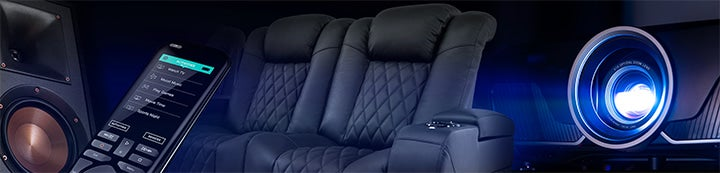1 - 2 of 2 Posts

mterle

·
Registered
Joined
·
1 Posts
Discussion Starter · ·
Ahhhh...I have officially taken the plunge. I did this 16 years ago with a turntable and it turned into a sickness. Anyway, I bought a fairly clean Sony last weekend and am wondering if someone can tell me what the throw distance is for a 16:9 at 72" wide screen. I tried opening up the throw distance calculator on curtpalme.com and had zero luck. Any help would be greatly appreciated. Also, does anyone know what term/s Sony uses in their set up manual for setting 'raster'.

Regards,

Matt

Shoujiki

·
Registered
Joined
·
168 Posts
Here's the calculations bud.

For a 72" diagonal wide screen,

First we need to find the width measurement for a 72" screen. This calculates to 62.77" for a 16:9 aspect ratio.

Then you must calculate for a non standard width screen as follows.

Screen size = Width (mm) x (5/4) / 25.4

So your width is 62.77", or 1594.3mm

So, 1594.3mm x (5/4) / 25.4 = 78.4".

So your screen is the equivalent of a 78.4" 4:3 screen, in terms of raster usage.

This means you use the SMALL CRT spacers and the SMALL Lens spacer setting, which may be hard to find. If you don't you will Max out the range on your electronic adjustments.

So now we have that, we use this formula to calculate throw distance.

Throw Distance = 25.78 x 78.4 + 191.

So for your 78.4" (4:3 equivalent screen) you will need a distance of 2.212 meters.

--------------------------------------------------------------------------------------

For a 72" wide, wide screen..

You must calculate for a non standard width screen as follows.

Screen size = Width (mm) x (5/4) / 25.4

So your width is 72", or 1828.8mm

So, 1828.8mm x (5/4) / 25.4 = ~90".

So your screen is the equivalent of a 90" 4:3 screen, in terms of raster usage.

This means you use the MEDIUM CRT spacers and the MEDIUM Lens spacer setting, which are the Sony standard ones.

So now we have that, we use this formula to calculate throw distance.

Throw Distance = 25.78 x 90 + 191.

So for your 90" (4:3 equivalent screen) you will need a distance of 2.511 meters.

1 - 2 of 2 Posts